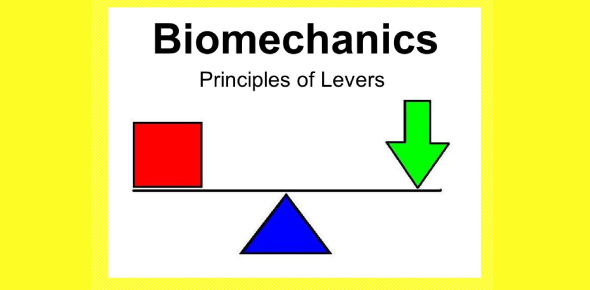# Basic Biomechanics And Levers

25 Questions | Total Attempts: 950SettingsThis quiz is designed to test basic knowledge over biomechanics and levers.

• 1.
The ­___________ is the point of pivot, which is also known as the axis of rotation.
• 2.
The _______________ is the distance from the weight to the fulcrum.
• 3.
A first order lever is like a __________, with the __________ between the in-force and the out-force.
• 4.
In a third order lever, the ___________ is closer to the fulcrum.
• 5.
In a second order lever, the ___________ is closer to the fulcrum.
• 6.
The most common kind of lever in nature is __________.
• 7.
The _________ order lever is the least common in nature.
• 8.
The equation for the balance of a lever deals with the _______ and the ________ of the lever arm.
• 9.
The length of the lever arm is perpendicular to the __________.
• 10.
The location of the fulcrum influences ___________ and __________.
• 11.
The _________ is perpendicular to the point of limb rotation.
• 12.
The _____________ is the perpendicular distance from the point of bone rotation to the line of muscle action.
• 13.
The out-lever is from the point of rotation to the point at which ___________ is applied.
• 14.
As the velocity ratio __________, speed decreases.
• 15.
As the velocity ratio decreases, force _________.
• 16.
The velocity ratio is the length of the ___________ divided by the length of the __________.
• 17.
A limb designed for force will have a _______________ velocity ratio than a limb designed for speed.
• 18.
Greater __________ causes a slower speed.
• 19.
Long lever arms sweep a ____________ distance, whereas short lever arms move a _____________ distance.
• 20.
__________ gear muscles enjoy a speed advantage and produce rapid limb oscillation.
• 21.
Unguligrades have the most speed because of an increased __________ or decreased ____________.
• 22.
A distally inserted muscle is better for __________ movements
• 23.
A ____________ inserted muscle is better for speed.
• 24.
The ____________ is an example of a low gear muscle.
• 25.
The mechanism of levers means that the output force and the output speed are ___________.
Related TopicsBack to top